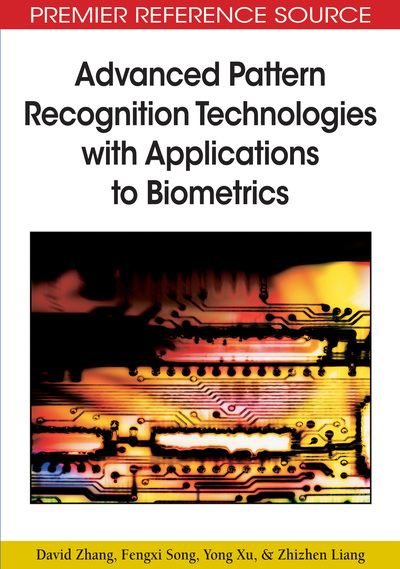# Tensor Linear Discriminant Analysis

David Zhang, Fengxi Song, Yong Xu, Zhizhen Liang
DOI: 10.4018/978-1-60566-200-8.ch009
OnDemand:
(Individual Chapters)
Available
\$29.50
No Current Special Offers

## Abstract

Linear discriminant analysis is a very effective and important method for feature extraction. In general, image matrices are often transformed into vectors prior to feature extraction, which results in the curse of dimensionality when the dimensions of matrices are huge. In this chapter, classical LDA and its several variants are introduced. In some sense, the variants of LDA can avoid the singularity problem and achieve computational efficiency. Experimental results on biometric data show the usefulness of LDA and its variants in some cases.
Chapter Preview
Top

## Introduction

Linear discriminant analysis is a popular technique for feature extraction, which has been successfully applied in many fields such as face recognition and character recognition. Linear discriminant analysis seeks to find the direction which maximizes between-class scatter and minimizes the within-class scatter. Based on linear discriminant analysis, Foley and Sammon (1975) proposed optimal discriminant vectors for two-class problems. Duchene and Leclercq (1988) further presented a set of discriminant vectors to solve multi-class problems. Although Foley-Sammon optimal discriminant vectors (FSODV) are orthogonal and perform well in some cases, the features which are obtained by optimal orthogonal discriminant vectors are statistically correlated. To avoid this problem, Jin, Yang, Hu, and Luo (2001) proposed a new set of uncorrelated discriminant vectors(UDV) which is proved to be more powerful than that of optimal orthogonal discriminant vectors in some cases. Then Jing, Zhang, and Jin (2003) further stated improvements on uncorrelated optimal discriminant vectors. Subsequently, Xu, Yang, and Jin (2003) studied the relationship between the Fisher criterion values of FSODV and UDV. Then Xu, Yang, and Jin (2004) developed a new model for Fisher discriminant analysis, which applies the maximal Fisher criterion and the minimal statistical correlation between features. Since the methods mentioned above are based on vectors rather than matrices, these methods face the computational difficulty when the dimension of data is too huge. To overcome this problem, Liu, Cheng, and Yang (1993) firstly proposed a novel linear projection method, which performs linear discriminant analysis in terms of image matrices. However, feature vectors using Liu’s method could be statistically correlated. In order to effectively deal with this problem, Yang, Yang, Frangi, and Zhang (2003) proposed a set of two-dimensional (2D) projection vectors which satisfy conjugate orthogonal constraints. Most importantly, feature vectors obtained by Yang’s method are statically uncorrelated. Then Liang, Shi, and Zhang (2006) proposed a new technique for 2D Fisher discriminant analysis. In their algorithm, the Fisher criterion function is directly constructed in terms of image matrices. Then they utilize the Fisher criterion and statistical correlation between features to construct an objective function. Then discriminant vectors are obtained in terms of the objection function. At the same time, they theoretically analyze that the proposed algorithm is equivalent to uncorrelated two-dimensional discriminant analysis in some condition. In Xiong, Swam and Ahmad (2005), one-sided 2DLDA is developed for classification tasks. Ye, Janardan, Park, and Park (2004) further developed generalized 2DLDA, which can overcome the singularity problem and achieves the computational efficiency. In Liang (2006; Yan et al., 2005), a multilinear generalization of linear discriminant analysis is discussed and an iterative algorithm is developed for solving multilinear linear discriminant analysis. Meanwhile, a non-iterative algorithm is also proposed in Liang (2006). In addition, multilinear LDA provides a unified framework for classical LDA and 2DLDA.

## Complete Chapter List

Search this Book:
Reset